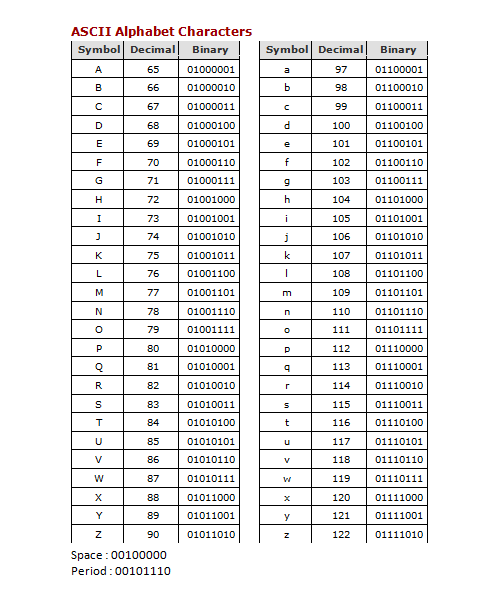# 2.3. It’s All Bits¶

## 2.3.1. Number Systems¶

The common decimal number system is based on ten numbers from 0 to 9. When we want to count higher than 9, we add additional digits to the left and multiply by ten each time. For example, in figure 1 below, we see a 9 in the ones position, a 7 in the tens position, a 1 in the hundreds position and a 2 in the thousands position. We would then multiply each digit by its position multiplier and add them all up to calculate our number: (2 * 1000) + (1 * 100) + (7 * 10) + (9 * 1) = 2000 + 100 + 70 + 9 = 2179

Computers use a binary number system, which means that everything is represented by two values: 0 and 1. These two values are combined to form letters, words, images, and everything else that shows up on your screen, but they also are used to represent states, such as on (1) or off (0) or the size of your RAM memory or magnetic disk.

We can also count to any number in binary, by combining the two numbers 0 and 1, just as we do in decimal. However, rather than multiplying by ten each time we add a number to the sequence, we multiply by two. For example, in figure 2 below, we see a 0 in the ones position, a 1 in the twos position, a 1 in the fours position, and a 0 in the eights position. We would then multiply each value by its position multiplier and add them all up to calculate our number: (0 * 8) + (1 * 4) + (1 * 2) + (0 * 1) = 0 + 4 + 2 + 0 = 6

You can try this with the below Binary Calculator. Go ahead and click on the box grey boxes in the twos and fours positions to verify that the calculator works correctly.

## 2.3.2. Bits and Bytes¶

Since the word digit comes from the practice of counting on our ten fingers and toes, early computer scientists needed another word to represent each 0 or 1 in a sequence. A bit or binary digit is a single 0 or 1 and is the smallest piece of information that a computer can store. A sequence of eight bits, such as 10010001 is called a byte. If you use the Binary Calculator above, you will see that the byte 10010001 is equal to 145.

Every time you save a document, image or video file, your computer writes a sequence of binary numbers to a storage device. You may be wondering how these 0’s and 1’s turn into numbers, letters, and words.

In essence, each item on your keyboard, be it a symbol, letter, or number is a character with a unique binary number. The American Standard Code for Information Interchange or ASCII, which was developed in 1963, portrays 95 characters with eight bits or one byte of information.

It gets more complicated when computers are representing media such as audio, video or images, but for the moment, it is enough to understand that all data on a computer is represented by 0s and 1s.Figure 3: ASCII Codes for Common Characters